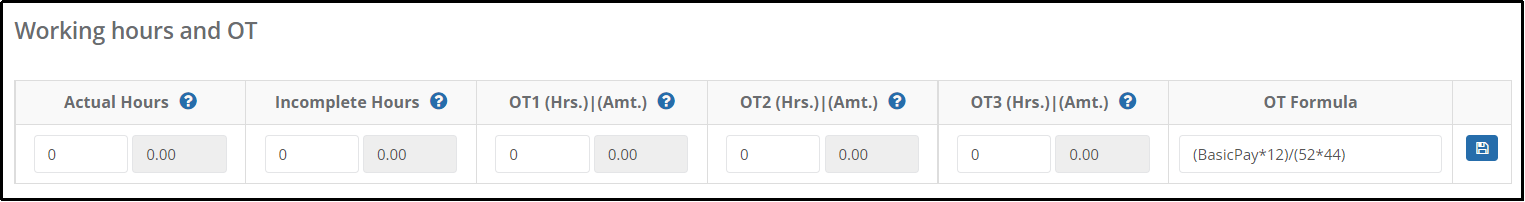# How are the Different OT Rates Defined in the Payroll Module?

This article will explain the different OTs in Payroll and how the system computes the values.

OT1 is applicable for working on PH (Public Holiday)

OT2 is applicable for rendering OT on a normal workday or working on Off Day.

OT3 is applicable for working on Rest Day.

Based on the rate defined, the OT amount will then reflect accordingly. Below is an example for clarity:

OT1 rate defined is 1.0; OT2 rate defined is 1.5; OT3 rate defined is 2.0

OT Formula: (BasicPay*12) / (52*44)

The OT amount that will be computed by the system will be as per below:

OT 1 (Sys) = ((BasicPay*12) / (52*44)) * OT1 Hours Worked * 1.0

OT 2 (Sys) = ((BasicPay*12) / (52*44)) * OT2 Hours Worked * 1.5

OT 3 (Sys) = ((BasicPay*12) / (52*44)) * OT3 Hours Worked * 2.0

If an employee is using a different OT formula, you may change accordingly in the Employee Pay Information page: